# Decision Making in Julia (if, if-else, Nested-if, if-elseif-else ladder)

• Last Updated : 19 Feb, 2020

Decision-making statements in programming languages decide the direction of the flow of program execution. A programming language uses control statements to control the flow of execution of a program based on certain conditions. These are used to cause the flow of execution to advance and branch based on changes to the state of a program.

Decision-making statements available in Julia are:

if statement

if statement is the most simple decision making statement. It is used to decide whether a certain statement or block of statements will be executed or not i.e if a certain condition is true then a block of statement is executed otherwise not.

Syntax:

```if condition
# Statements to execute if
# condition is true
end
```

Here, conditions after evaluation will be either true or false. if-statement accepts boolean values – if the value is true then it will execute the block of statements below it otherwise not. We can use condition with bracket ‘(‘ ‘)’ also.
Statements written within the if-statement and the end statement are considered as a block and are executed if the condition is satisfied.

Flowchart:-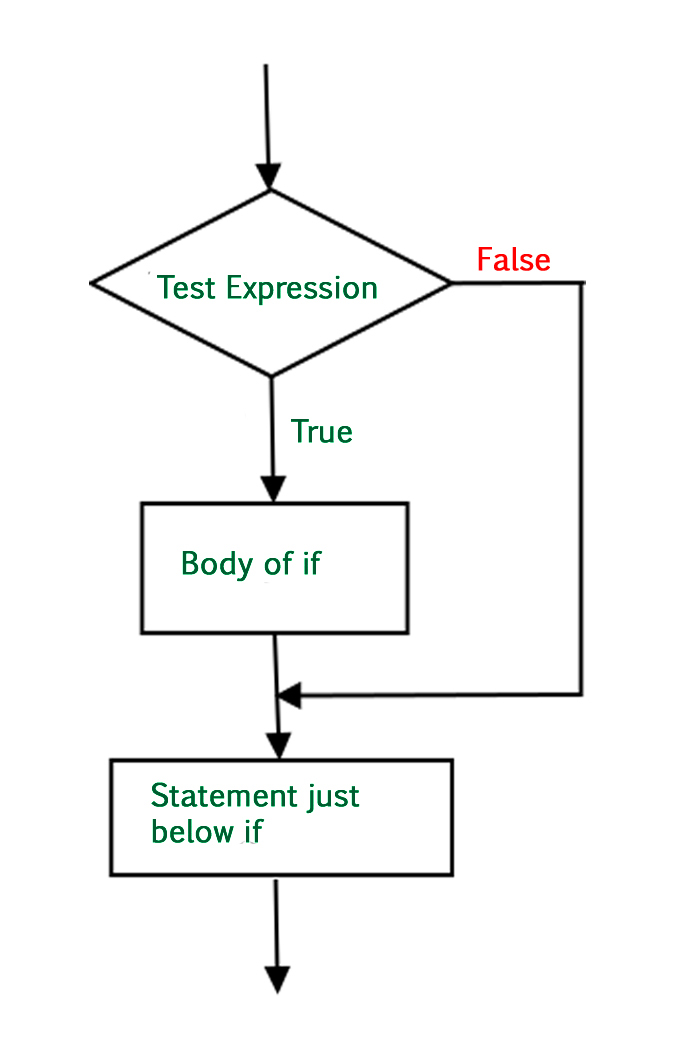`# Julia program to illustrate If statement`` ` `i ``=` `10``if` `(i > ``15``)``   ``println(``"10 is greater than 15"``)``end``println(``"I am Not in if"``)`

Output: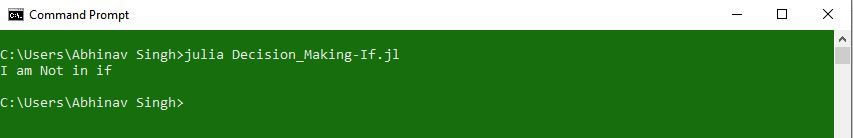In the above code, the condition present in the if-statement is false. So, the block below the if-statement is not executed.

if- else

The if statement alone tells us that if a condition is true it will execute a block of statements and if the condition is false it won’t. But what if we want to do something else if the condition is false. Here comes the else statement. We can use the else statement with if statement to execute a block of code when the condition is false.
Syntax:

```if (condition)
# Executes this block if
# condition is true
else
# Executes this block if
# condition is false
end
```

Flow Chart:-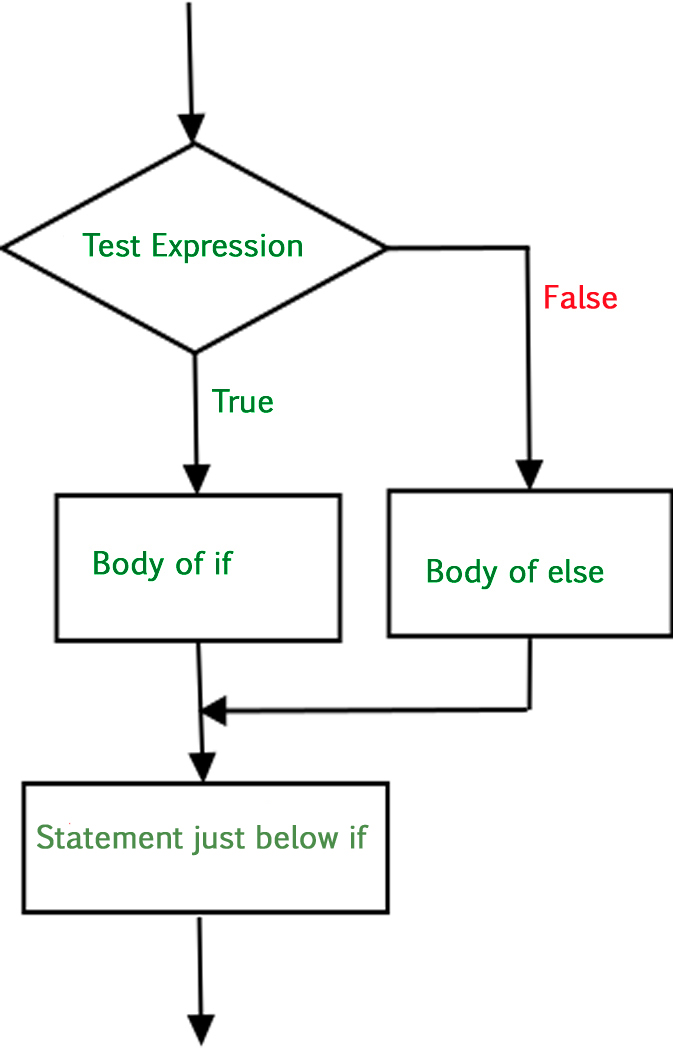`# Julia program to illustrate If-else statement`` ` `i ``=` `20``;``if` `(i < ``15``)``    ``println(``"\$i is smaller than 15"``)``    ``println(``"I'm in if Block"``)``else``    ``println(``"\$i is greater than 15"``)``    ``println(``"I'm in else Block"``)``end``println(``"I'm not in if and not in else Block"``)`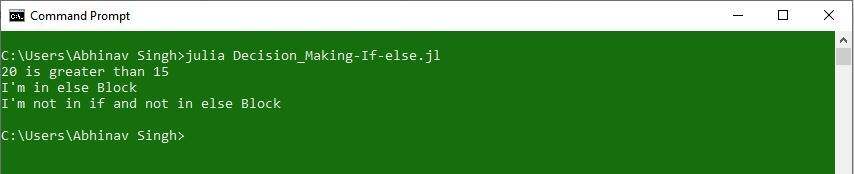In the above code, the condition given in the if-statement is false. Hence, the block of code written in the else-statement is executed. Afterwards, when the execution of the else block is over, the compiler executes the statement written outside of the If-else statement.

nested-if

A nested-if is an if-statement that is the target of another if-statement. Nested-if statements means an if-statement written inside another if-statement. Yes, Julia allows us to nest if-statements within if-statements. i.e, we can place an if-statement inside another if-statement and so on multiple if-statements can be used as per the need.

Syntax:

```if (condition1)
# Executes when condition1 is true
if (condition2)
# Executes when condition2 is true
# if Block ends here
end
# if Block ends here
end
```

Flow chart:-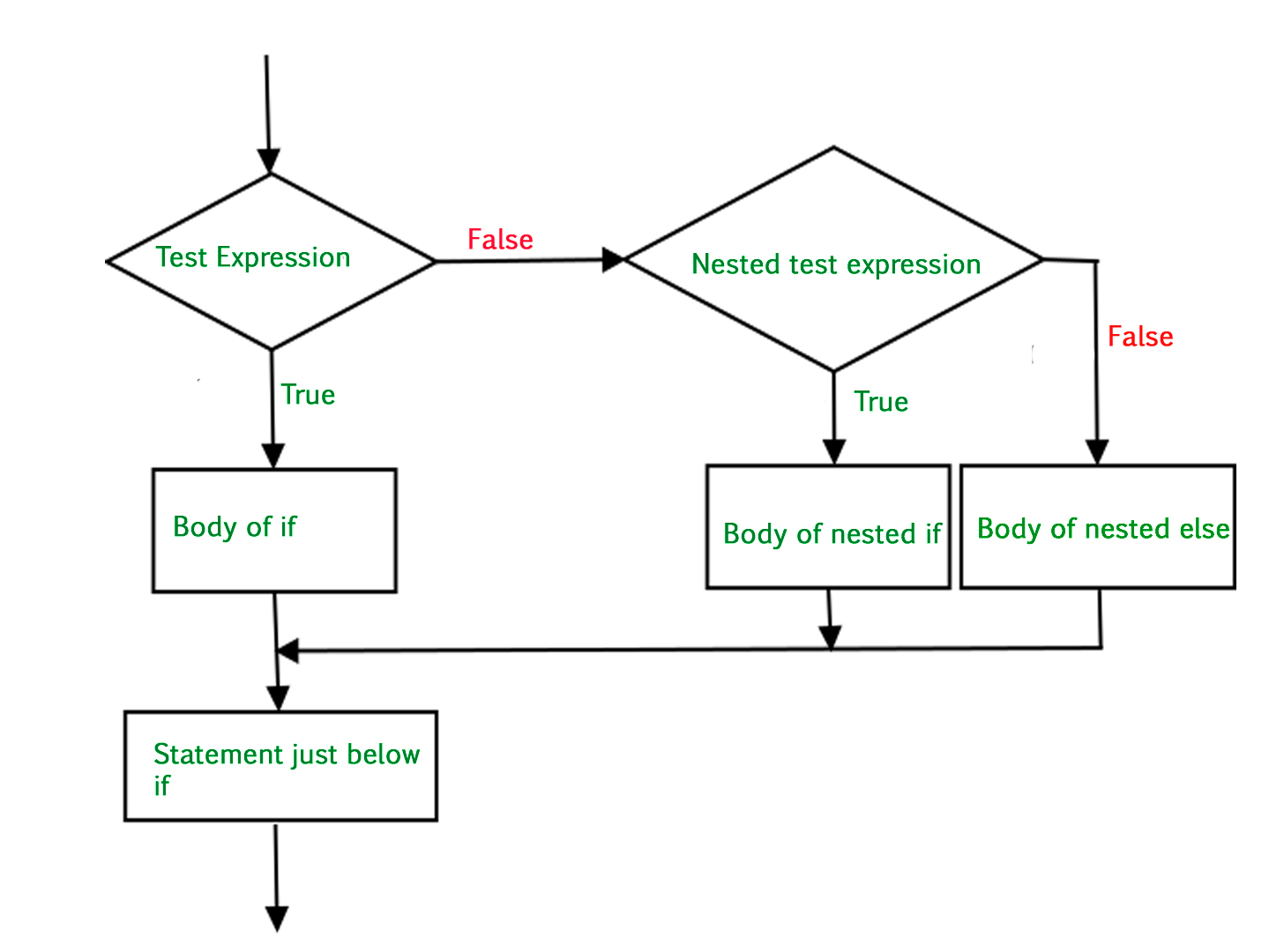`# Julia program to illustrate nested-If statement`` ` `i ``=` `14`` ` `# First if statement``if` `(i ``=``=` `14``)`` ` `    ``# First Nested-if statement``    ``if` `(i < ``15``)``        ``println(``"\$i is smaller than 15"``)`` ` `        ``# This Nested statement``        ``# will only be executed if ``        ``# the first Nested-if statement is true``        ``if` `(i < ``12``)``            ``println(``"\$i is smaller than 12 too"``)``        ``else``            ``println(``"\$i lies between 12 and 15"``)``        ``end``    ``else``        ``println(``"\$i is greater than 15"``)``    ``end``end`

Output: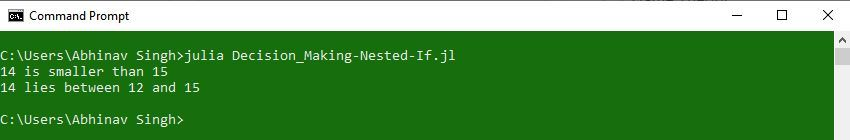Here, a user can decide among multiple options. The if-statements are executed from the top down. As soon as one of the conditions controlling the if is true, the statement associated with that if is executed, and the rest of the ladder is bypassed. If none of the conditions is true, then the final else statement will be executed.

Syntax:

```if (condition)
statement
elseif (condition)
statement
.
.
else
statement
end
```

Flow Chart:-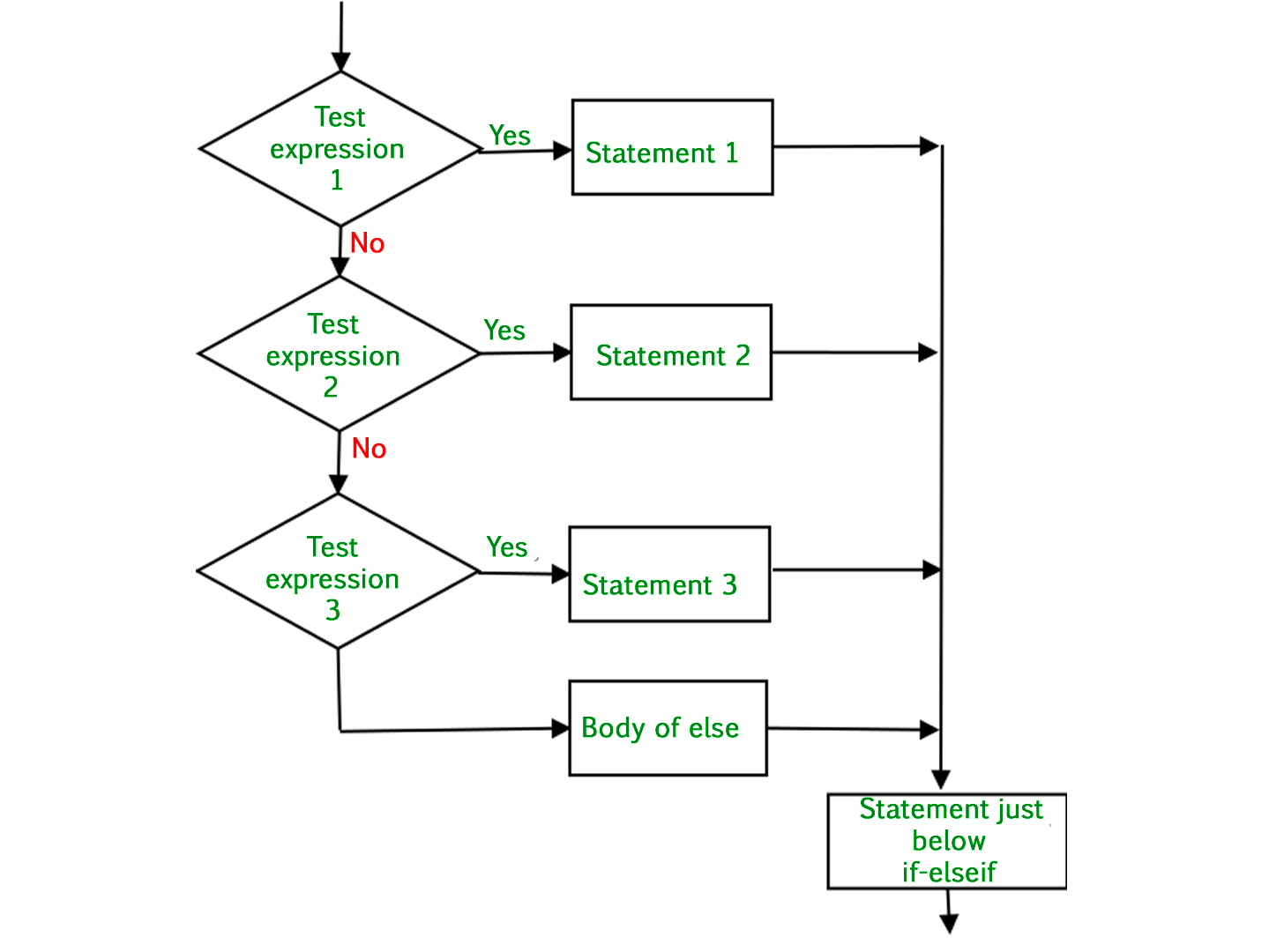`# Julia program to illustrate if-elseif-else ladder`` ` `i ``=` `20``if` `(i ``=``=` `10``)``    ``println(``"Value of i is 10"``)``elseif(i ``=``=` `15``)``    ``println(``"Value of i is 15"``)``elseif(i ``=``=` `20``)``    ``println(``"Value of i is 20"``)``else``    ``println(``"Value of i is not defined"``)``end`

Output: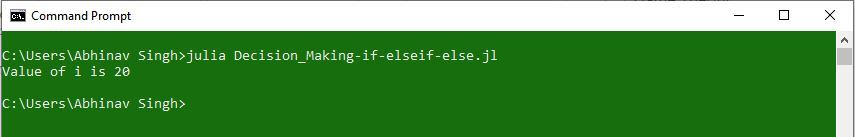My Personal Notes arrow_drop_up